Chapter 5, Problem 5.9EP### Organic And Biological Chemistry

7th Edition
STOKER + 1 other
ISBN: 9781305081079

#### Solutions

Chapter
Section### Organic And Biological Chemistry

7th Edition
STOKER + 1 other
ISBN: 9781305081079
Textbook Problem

# Classify each of the compounds in Problem 16-7 as a carbonyl compound or an acyl compound.a.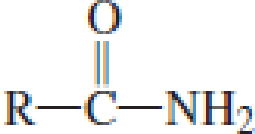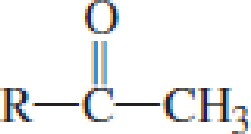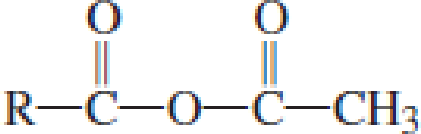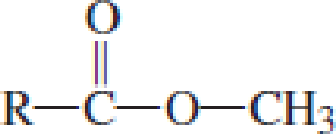(a)

Interpretation Introduction

Interpretation:

The given compound is a carbonyl compound or acyl compound has to be classified.

Concept Introduction:

Carbonyl groups are the one which contain a double bond between carbon and oxygen atom.  Aldehydes and ketones possess this carbonyl functional group in it.  The structural representation of a carbonyl group can be given as shown below,If a hydroxyl group is attached to a carbonyl group means it is known as carboxyl group.  This can be represented as shown below,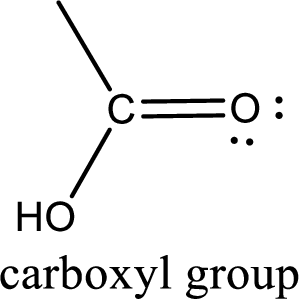Carboxylic acid derivatives are the ones that are synthesized from or converted to a carboxylic acid.  The generalized structural representation of carboxylic acid derivatives is shown below,Carbon bonded to an oxygen atom through double bond is known as carbonyl group.  The carbonyl group bonded to a R group means it is known as acyl group.  If the acyl group is bonded to oxygen, nitrogen or halogen atom then the compound is known as acyl compound.  If the acyl group is bonded to a carbon or hydrogen atom, then the compound is known as carbonyl compound.

Explanation

The general structure of compound is,

The acyl group is the carbonyl group attached to a R group...

(b)

Interpretation Introduction

Interpretation:

The given compound is a carbonyl compound or acyl compound has to be classified.

Concept Introduction:

Carbonyl groups are the one which contain a double bond between carbon and oxygen atom.  Aldehydes and ketones possess this carbonyl functional group in it.  The structural representation of a carbonyl group can be given as shown below,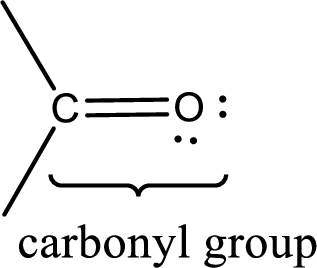If a hydroxyl group is attached to a carbonyl group means it is known as carboxyl group.  This can be represented as shown below,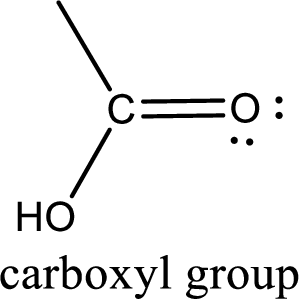Carboxylic acid derivatives are the ones that are synthesized from or converted to a carboxylic acid.  The generalized structural representation of carboxylic acid derivatives is shown below,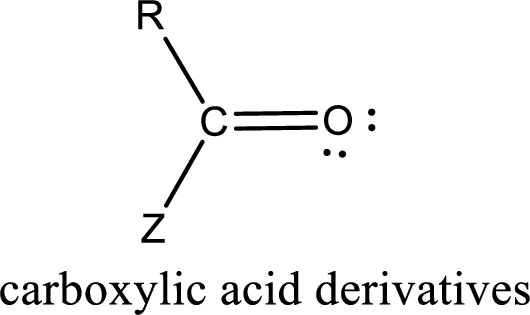Carbon bonded to an oxygen atom through double bond is known as carbonyl group.  The carbonyl group bonded to a R group means it is known as acyl group.  If the acyl group is bonded to oxygen, nitrogen or halogen atom then the compound is known as acyl compound.  If the acyl group is bonded to a carbon or hydrogen atom, then the compound is known as carbonyl compound.

(c)

Interpretation Introduction

Interpretation:

The given compound is a carbonyl compound or acyl compound has to be classified.

Concept Introduction:

Carbonyl groups are the one which contain a double bond between carbon and oxygen atom.  Aldehydes and ketones possess this carbonyl functional group in it.  The structural representation of a carbonyl group can be given as shown below,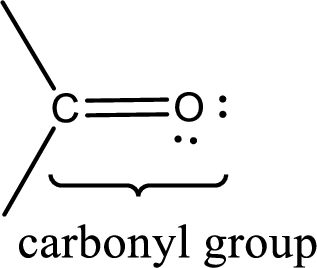If a hydroxyl group is attached to a carbonyl group means it is known as carboxyl group.  This can be represented as shown below,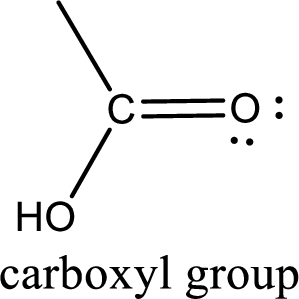Carboxylic acid derivatives are the ones that are synthesized from or converted to a carboxylic acid.  The generalized structural representation of carboxylic acid derivatives is shown below,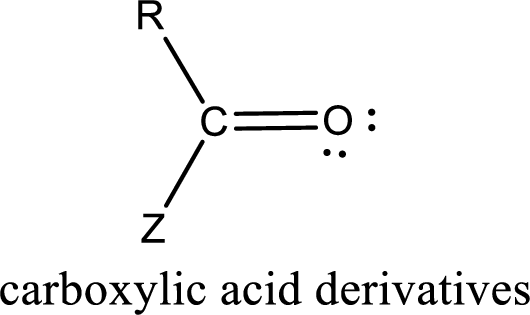Carbon bonded to an oxygen atom through double bond is known as carbonyl group.  The carbonyl group bonded to a R group means it is known as acyl group.  If the acyl group is bonded to oxygen, nitrogen or halogen atom then the compound is known as acyl compound.  If the acyl group is bonded to a carbon or hydrogen atom, then the compound is known as carbonyl compound.

(d)

Interpretation Introduction

Interpretation:

The given compound is a carbonyl compound or acyl compound has to be classified.

Concept Introduction:

Carbonyl groups are the one which contain a double bond between carbon and oxygen atom.  Aldehydes and ketones possess this carbonyl functional group in it.  The structural representation of a carbonyl group can be given as shown below,If a hydroxyl group is attached to a carbonyl group means it is known as carboxyl group.  This can be represented as shown below,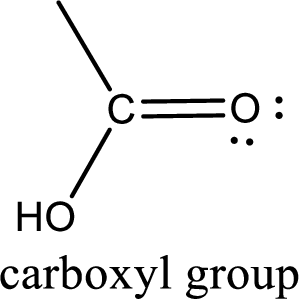Carboxylic acid derivatives are the ones that are synthesized from or converted to a carboxylic acid.  The generalized structural representation of carboxylic acid derivatives is shown below,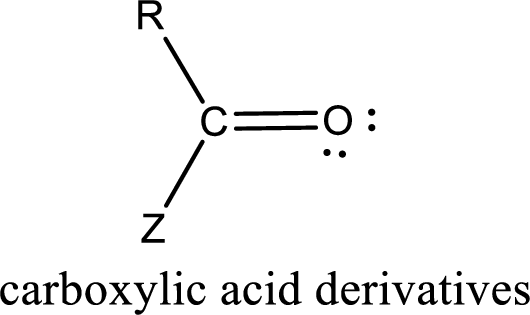Carbon bonded to an oxygen atom through double bond is known as carbonyl group.  The carbonyl group bonded to a R group means it is known as acyl group.  If the acyl group is bonded to oxygen, nitrogen or halogen atom then the compound is known as acyl compound.  If the acyl group is bonded to a carbon or hydrogen atom, then the compound is known as carbonyl compound.

### Still sussing out bartleby?

Check out a sample textbook solution.

See a sample solution

#### The Solution to Your Study Problems

Bartleby provides explanations to thousands of textbook problems written by our experts, many with advanced degrees!

Get Started